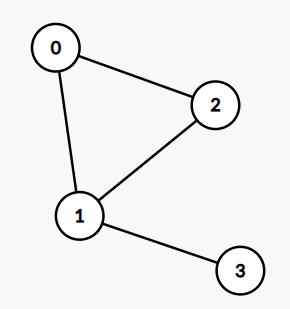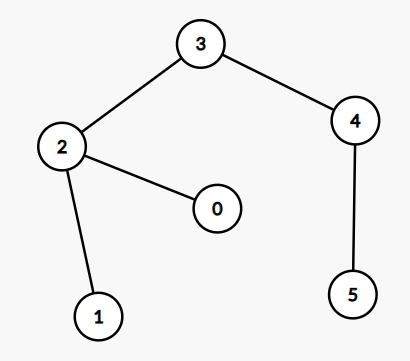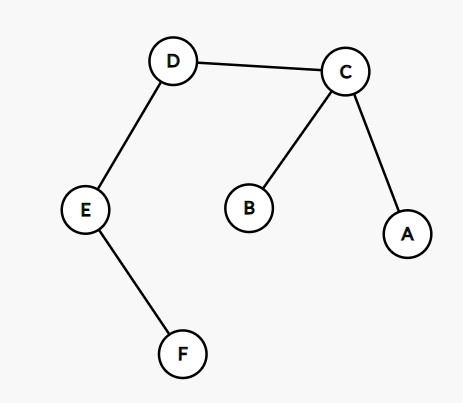# Pendant Vertices, Non-Pendant vertices, Pendant Edges, and Non-Pendant Edges of the Graph.

## Introduction

In this article, we will discuss about pendant vertices, non-pendant vertices, pendant edges, and non-pendant edges of the graph. We will first read the formal definition and then visualize it using the graph.

### Degree Of the Vertex in a graph

The degree of the vertex in a graph is defined as the number of edges connected to the vertex.In the given Graph,

Node 0 has 2 edges connected to it. Therefore it has degree 2.

Node 1 has 3 edges connected to it. Therefore it has degree 3.

Node 2 has 2 edges connected to it. Therefore it has degree 2.

Node 3 has 1 edge connected to it. Therefore it has degree 1.

## Pendant Vertices

In a given graph, Let say G, for any vertice to be a pendant vertex, the degree of the vertex should be 1. In the case of the tree, the pendant vertex is known as leaf or leaf nodes or terminal nodes because in the case of trees leaf node always has degree 1.

In the given graph, identify the pendant vertices:Node 0 has one edge. Therefore it has degree 1.

Node 1 has 1 edge. Therefore it has degree 1.

Node 2 has 3 edges. Therefore it has degree 3.

Node 3 has two edges. Therefore it has degree 2.

Node 4 has two edges. Therefore it has degree 2.

Node 5 has one edge. Therefore it has degree 1.

So pendant vertices are nodes with degree 1.

These are Node 0, Node 1, and Node 5.

### Implementation in C++

``````// C++ program for the above approach
#include <bits/stdc++.h>
using namespace std;

// Function to print all the pendant
// vertices
void printPendantVertices(  map<int, vector<int>> G)
{

// iterating over all the edges for any node
// if the size of vector edge is 1, then it must be pendant vertex
for (auto it=G.begin();it!=G.end();it++) {
if (it->second.size() == 1) {
cout << "Node" << it->first << endl;
}
}
}

void insertIntoGraph(map<int,vector<int>>&G,int x,int y){
G[x].push_back(y);
G[y].push_back(x);
return;
}
// Driver Code
int main()
{

map<int, vector<int>> G;
int edges = {{1,2}, {2,0}, {2,3}, {3,4}, {4,5}};
for(int i=0;i<5;i++){
insertIntoGraph(G, edges[i], edges[i]);
}
printPendantVertices(G);

return 0;
}

Output :
Node0
Node1
Node5``````

## Non-Pendant Vertices

In a given graph, Let say G, Non-pendant vertices are those vertices that are non - pendant, i.e., the degree should be greater than 1. In the case of trees, non-pendant vertices are non-leaf nodes because it does not have degree 1.

In the given graph, identify the non-pendant vertices:Node 0, has one edge. Therefore it has degree 1.

Node 1 has one edge. Therefore. Therefore it has degree 1.

Node 2 has three edges. Therefore it has degree 3.

Node 3 has two edges. Therefore it has degree 2.

Node 4 has two edges. Therefore it has degree 2.

Node 5 has one edge. Therefore it has degree 1.

So non-pendant vertices are nodes with degrees greater than 1.

These are Node 2, Node 3, and Node 4.

## Pendant Edges

In a given graph, Let say G, The edge is a pendant edge if and only if at least of the vertices joining the edges is a pendant vertex.

In the given graph, identify the pendant edges:Node A has one edge, therefore it has degree 1.

Node B has one edge. Therefore it has degree 1.

Node C has three edges. Therefore it has degree 3.

Node D has two edges. Therefore it has degree 2.

Node E has two edges. Therefore it has degree 2.

Node F has one edge. Therefore it has degree 1.

Edges between pendant vertices are pendant edges. Therefore pendant edges are AC, BC, EF.

## Non-Pendant Edges

In a given graph, Let say G, The edge is said to be a  non-pendant edge if and only if it does not contain a pendant vertex as one of its vertices.

In the given graph, identify the non-pendant edges:Node A has one edge. Therefore it has degree 1.

Node B has one edge. Therefore it has degree 1.

Node C has three edges. Therefore it has degree 3.

Node D has two edges. Therefore it has degree 2.

Node E has two edges. Therefore it has degree 2.

Node F has one edge. Therefore it has degree 1.

Edges between the vertices whose degree is greater than one are non-pendant edges.

Therefore non-pendant edges are DC, DE.

## Practice Problem

Prerequisites: Handshaking Theorem

Ques. Suppose that a tree X has 4 vertices of degree 3, 2 vertices of degree 2, and 3 vertices of degree 4. find the number of pendant vertices in tree X?

Solution. According to the Handshaking Theorem,

Sum of all degrees = 2*(Number of edges).

We Know, in Tree Number of edges = number of vertices - 1.

In this Given Tree X, the Total number of vertices = 4 + 2 + 3 + k, where k is the number of vertices with degree 1.

Total Edges = 4 + 2 + 3 + k - 1.

According to the Handshaking formula

Sum of all degrees = 2*(number of edges)

4*3 + 2*2 + 3*4 + k*1 = 2*(4+2+3+k -1)

Solving the equation we get, k = 12.

Therefore the total number of pendant vertices is 12.

Q1. How many edges are possible in a graph with N vertices?

Ans. In a graph with N vertices, an edge can connect with N-1 other vertices, so In a directed graph, a total number of possible edges is N*(N-1) and in case of undirected graph total number of edges is N*(N-1)/2.

Q2. What is the handshaking theorem in a graph?

Ans. According to the handshaking theorem, the number of degrees in a graph is equal to 2*(number of edges in the graph).

Q3. What are the ways to represent the graph and which one is more efficient?

Ans. Graphs can be represented as Adjacency list and adjacency matrix. If the number of edges is more, then the adjacency matrix is good otherwise adjacency list is a good method.

## Key takeaways

In this article, We have discussed about pendant vertices, non-pendant vertices, pendant edges, and non-pendant edges in a graph. We hope you understand these topics properly of graph theory. If you are a beginner, interested in coding, and want to learn DSA, you can look for our guided path for DSA, which is free!